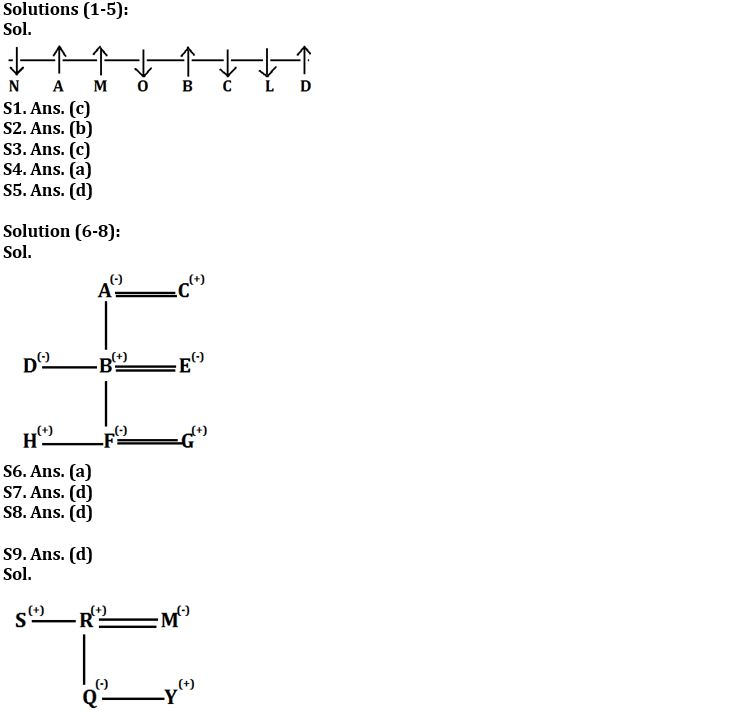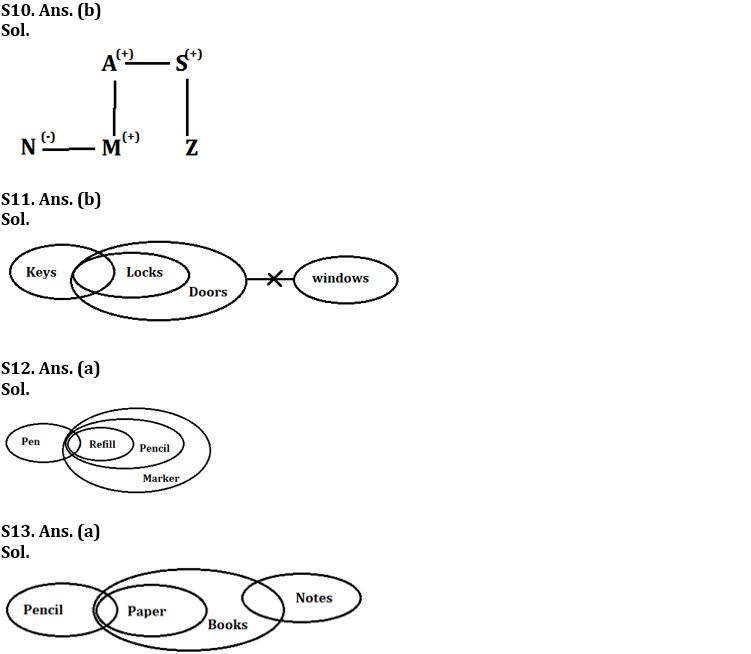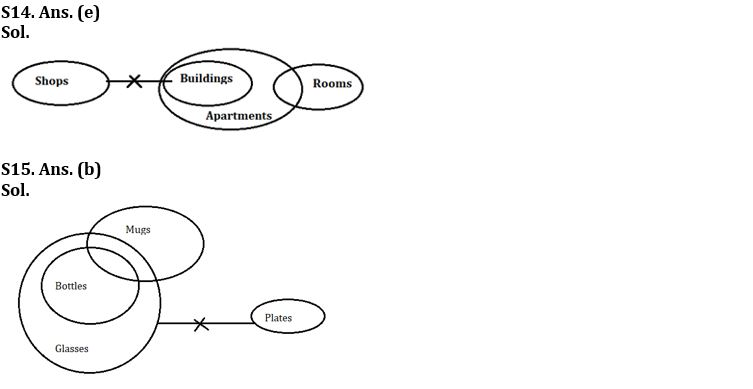Latest Banking jobs   »

# Reasoning Ability Quiz For IBPS Clerk/PO Prelims 2022- 10th September

Directions (1-5): Study the following information and answer the questions.
Eight friends L, M, N, O, A, B, C and D are seated in a straight line, but not necessarily in the same order. Some of them are facing north and some are facing south. A is an immediate neighbour of one who is sitting at an extreme end of the line. Only three people sit between A and C. O sits second to the right of C. O does not sit at an extreme end of the line. D sits on the immediate left of L. D is not an immediate neighbour of C. The immediate neighbours of L face opposite direction. The persons who are sitting at the extreme ends face opposite directions. M sits second to the left of B. B faces north. B is not an immediate neighbour of A. The immediate neighbours of B face same direction. Both A and M face a direction opposite to that of O.

Q1. As per the given arrangements, which of the following statements is not true with respect to L?
(a) L faces south.
(b) L is fourth to the right of M.
(c) L is 2nd to left of A.
(d) L is between C and D.
(e) None of these

Q2. How many persons sit on the left of M?
(a) One
(b) Two
(c) Three
(d) Four
(e) None of these

Q3. What is the position of O with respect to L?
(a) Immediate left
(b) Third to left
(c) Third to right
(d) Fourth to left
(e) None of these

Q4. Who among the following are immediate neighbours of M?
(a) A, O
(b) A, B
(c) C, B
(d) N, O
(e) None of these

Q5. Four of the given five are alike in a certain way based on the given arrangement and hence form a group. Who among the following does not belong to that group?
(a) N
(b) O
(c) L
(d) B
(e)C

Directions (6-8): Study the following information carefully to answer the following question:
There are eight members in the family having three generations. There are only three married couple. A is mother of D. G is son in law of B. H is nephew of D. C has only one son. F is granddaughter of C. E is mother of F. D is unmarried.

Q6. How C is related to D?
(a) Father
(b) Mother
(c) Son
(d) Son in law
(e) Brother

Q7. Who among the following is son in law of E?
(a) A
(b) C
(c) H
(d) G
(e) None of these

Q8. Who among the following is niece of D?
(a) C
(b) E
(c) H
(d) F
(e) G

Directions (9-10): Each of these questions is based on the following information:
(i) P % Q means P is the father of B.
(ii) P @ Q means P is the sister of B.
(iii) P \$ Q means P is the brother of B.
(iv) P * Q means P is the son of B.

Q9. In the expression ‘S \$ R % Q @ Y * M’, how is M related to S?
(a) Aunt
(b) Mother
(c) Sister
(d) Sister-in-law
(e) None of these

Q10. In the expression N @ M * A \$ S % Z, how is S related to N?
(a) Aunt
(b) Uncle
(c) Sister
(d) Sister-in-law
(e) None of these

Directions (11-15): Each question consists of three statements followed by two conclusions numbered I and II. Consider the given statements to be true even if they seem to be at variance with commonly known facts. Read all the conclusions and then decide which of the given conclusion logically follow from the given statements using all statements together. Mark your answer as,
(a) If only conclusion I follows
(b) If only conclusion II follows
(c) If either conclusion I or conclusion II follows
(d) If neither conclusion I nor conclusion II follows
(e) If both conclusion I and conclusion II follow

Q11. Statements: Some Keys are locks.
All locks are doors
No doors are windows
Conclusions: I. Some doors are keys is a possibility.
II. Some windows are not locks.

Q12. Statements: Some pen are refill.
All refill are pencil
All pencil are marker
Conclusions: I. Some pen are marker.
II. Some marker can be refill.

Q13. Statements: Only a few pencil is a paper
All papers are books
Some books are notes
Conclusions: I. Some Paper are notes is a possibility.
II. Some books are not Pencil.

Q14. Statements: No shops are buildings
All buildings are apartments
Only a few apartments are rooms
Conclusions: I. All rooms can be building.
II. Some apartments are not shops.

Q15. Statements: All Bottles are glasses
Only a few mugs are Bottles
No glasses are plates
Conclusions: I. Some bottles are not plates is a possibility.
II. Some glasses are bottles.

Solutions#### Congratulations!Download Hindu Review of October 2021: Free PDF